# Online Convex Optimization

Disclaimer: we will require some familiarity with convex optimization.

## 1 What game are we playing?

The player is given a fixed compact convex decision set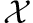. At each round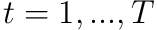:

1. Player pick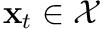.
2. The environment picks a convex loss function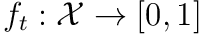.
3. The player then gets access to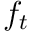and suffers loss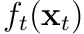.

We want to have some notion of whether we are doing well. In the above setting simply using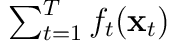we could never do well since the environment can pick a new adversarial functionevery time. Instead we compare ourselves with the best fixed strategy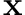:

Regret is defined asThis is called the regret as it captures how badly we did in hindsight Ideally we want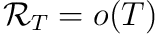since then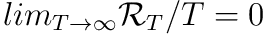.

.

To get a feel let’s first try a naive approach. A straight forward idea is what goes by the name of Follow The Leader (FTL) in the machine learning community and ficticious play in economics. We picksHowever, there is a simple adversarial strategy against this algorithm for the environment. Consider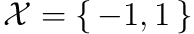then the environment picksSo when minimizing the following at every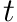we haveWe will end up always picking the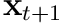that leads to the maximum regret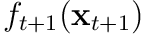. So we achieve the lower bound of the regret. Can we do better?

### 2.1 Be The Leader (BLT)

Let’s say that we could cheat and playat time— thus avoiding the adversary. For notational convenience we still keep the same definition:However, now in the regret analysis we incur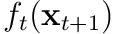instead of. We can now redo the regret analysis:Not surprisingly BTL’s regret is negative. But it provides an interesting insight:The above is just a simplification of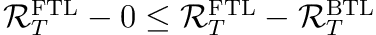which is immediately obtained by application of the BTL bound.

So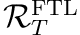is no worse than the difference betweenand. Intuitively a solution to our problem with FTL is then to make sure the strategy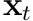does not change too much — in other words: we want to regularize / stabilize!

## 3 Follow The Regularized Leader (FTRL)

We modify our selectionwhere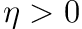is the learning rate and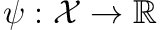is a regularizer.

The regularizer is there to ensure that our choice does not change too much between iterations. To capture this we will rely on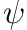being strongly convex. That is,for some norm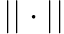.

### 3.1 Stability

This will allow us to prove the following notion of stability.

Stability says that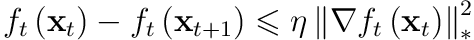with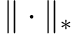is the dual norm of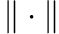defined as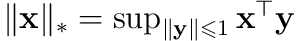.

Let’s define the function we minimizes in the decision selectionBy strong convexity ofand convexity of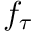we have that This will ultimately let us bound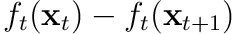.RFTL defines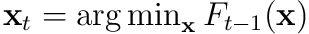so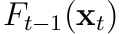is the optimum and first order characterizationFirst order characterization of convexity says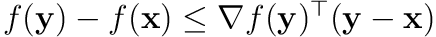. So when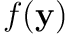is minimum we have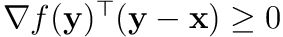.

becomes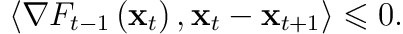So a weaker bound of eq. 1 isThe second line simply applies the same reasoning. We can now sum the two lines and expand the definition of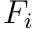. All terms will cancel out except the last term in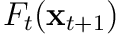and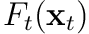so we getNow we have the tools to bound the change Hölder’s inequality: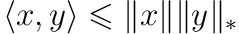.

.Solving forwe get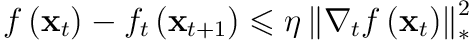Equipped with stability and the BTL lemma we almost directly obtain the regret bound for FTRL.

### 3.2 Regret bound for FTRL

With learning rate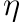we havewhere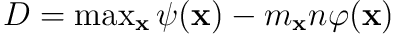.

By defining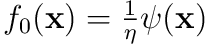we can write the regularized selection asFirst note that by the BTL lemma we haveNote that we additionally use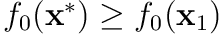since BTL only applies to.withNow we can bound the FTRL regret as followsWe want to use stability so we need to move from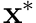to. We do this by getting it on a form on which we can apply the BTL lemma.Further, if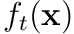is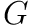-Lipschitz constraint (so that the gradient is bounded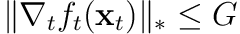) and the optimal learning rateThe optimalis found by simply optimizing the above regret bound which is of the form: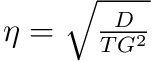is chosen, then we have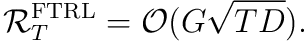## 4 FTRL with linear losses

At this point we have good regret bounds but at every iterationwe have to minimize a sum ofconvex functions. This simplifies significantly if we assume that the loss is linear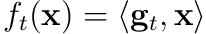since we can push in the summation:Now we only have to keep track of a sum of gradients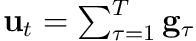and the minimization problem reduces to a sum of a linear function and the strongly convex regularizer. So we can write the program as:

FTRL with linear loss

1.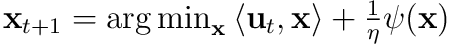2.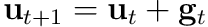### 4.1 Linear Loss is Sufficient

It turns out that we can assume linear loss without loss of generality! That is, we can get the same regret bound for convex loss functions even if we run the above algorithm only using the gradient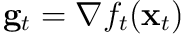at each. To see this, considerSo we can bound the regret forby bounding the regret for the new problem which only considers its gradient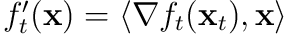. The bound stays as tight since we already used convexity in our argument for stability.

These notes are primarily based on Prof. Luo’s lectures notes  with minor modifications borrowed from Hazan’s book  and the lecture notes of Prof. Orabona .

 “CSCI 699: Introduction to Online Learning.” [Online]. Available: https://haipeng-luo.net/courses/CSCI699/. [Accessed: 02-Nov-2019]

 E. Hazan, “Introduction to Online Convex Optimization,” Sep. 2019 [Online]. Available: http://arxiv.org/abs/1909.05207. [Accessed: 02-Nov-2019]

 “Introduction to Online Learning,” Parameter-free Learning and Optimization Algorithms, 20-Sep-2019. [Online]. Available: https://parameterfree.com/lecture-notes-on-online-learning/. [Accessed: 02-Nov-2019]